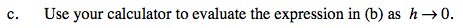### Home > AC > Chapter 16 > Lesson 16.9.2.1 > Problem9-69

9-69.
1. Let g(x) =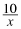. Homework Help ✎

1. Find the average rate of change of g(x) from x = 5 to x = 6.

2. Write the expression for the average rate of change of g(x) from x = 5 to x = 5 + h.

3. Use your calculator to evaluate the expression in (b) as h → 0.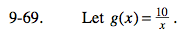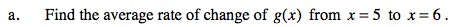$\frac{\frac{10}{6}-\frac{10}{5}}{6-5}$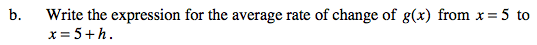$\frac{\frac{10}{5+h}-\frac{10}{5}}{5+h-5}$